# vs.eyeandcontacts.com

## Chapter 4 Practical Geometry Exercise 4.2

Question 1: Construct the following quadrilaterals.
LI = 4 cm
IF = 3 cm
TL = 2.5 cm
LF = 4.5 cm
IT = 4 cm
OL = 7.5 cm
GL = 6 cm
GD = 6 cm
LD = 5 cm
OD = 10 cm
iii) Rhombus BEND
BN = 5.6 cm
DE = 6.5 cm

i)
Given
LI = 4 cm
IF = 3 cm
TL = 2.5 cm
LF = 4.5 cm
IT = 4 cm

Rough figure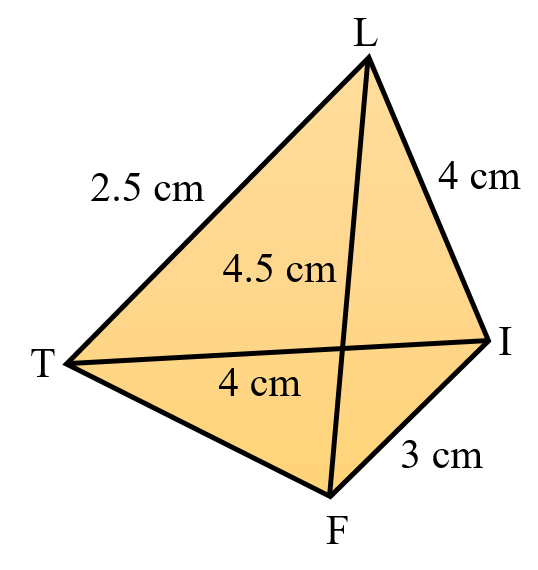Actual figure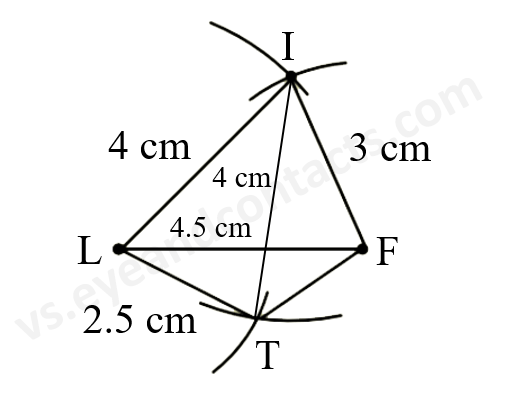Steps of Construction
1. Draw line segment LF = 4.5 cm.
2. With L as centre, radius 4 cm draw an arc.
3. With F as radius, radius 3 cm draw an arc to cut previous arc at I.
4. With L as centre, radius 2.5 cm draw an arc.
5. With I as centre, radius 4 cm draw an arc to cut previous arc at T.
6. Join LT, LI, TF, FT.
7. LIFT is the required quadrilateral.

ii)
Given
OL = 7.5 cm
GL = 6 cm
GD = 6 cm
LD = 5 cm
OD = 10 cm

Rough figure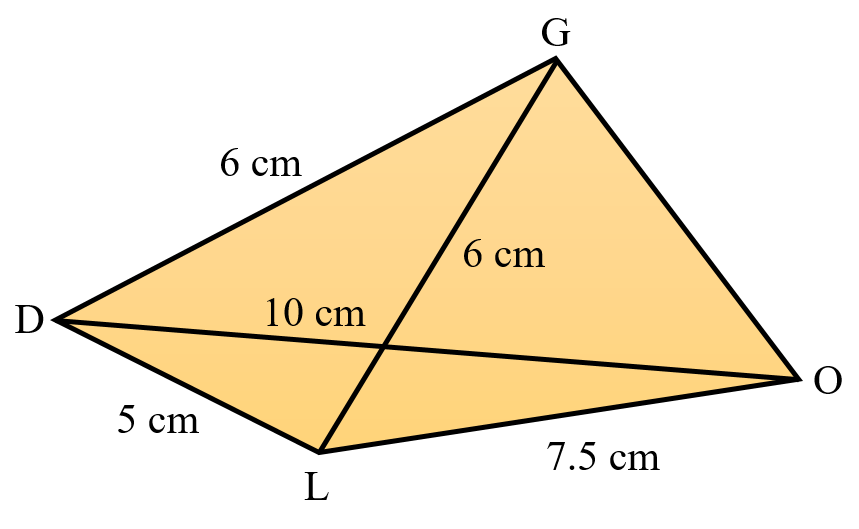Actual figure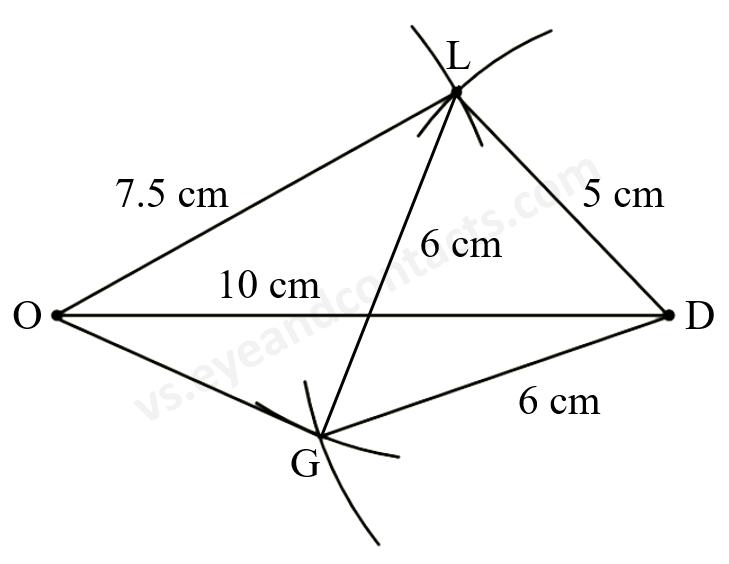Steps of Construction
1. Draw line segment OD = 10 cm.
2. With O as centre, radius 7.5 cm draw an arc.
3. With D as centre, radius 5 cm draw an arc to cut previous arc at L.
4. With D as centre, radius 6 cm draw an arc.
5. With L as centre, radius 6 cm draw an arc to cut the previous arc at G.
6. Join OL, DL, OG, DG.
7. GOLD is the required quadrilateral.

iii)
Given
Rhombus BEND
BN = 5.6 cm
DE = 6.5 cm

Rough figure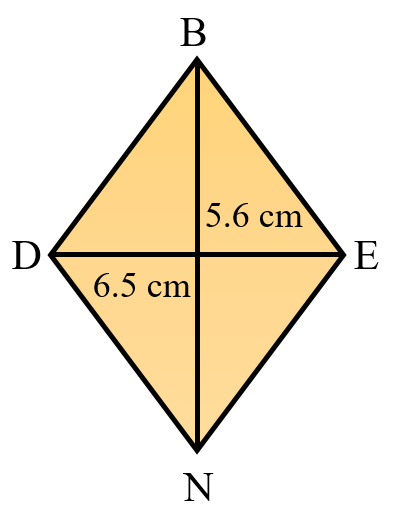Actual figure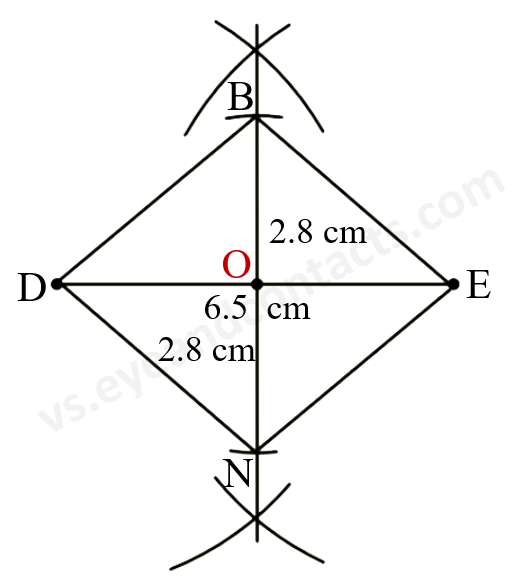Steps of Construction
1. Draw line segment DE = 6.5 cm.
2. Draw the perpendicular bisector of DE to intersect DE at O (O is the midpoint of DE).
3. With O as the centre and radius = 2.8 cm, draw arcs on either sides to intersect the perpendicular bisector at B and N.
4. Join BD, BE, DN, EN.
5. BEND is the required rhombus.